### Overview of neural network architectures

(This article was initially in the form of several disparate articles, which I've compiled into one because they were rather short and trivial on their own, and didn't communicate significant insights.)

Below is a list of some basic "features" of human cognition that come to mind upon a cursory introspection:
• Data type specific processing: the brain has specific mechanisms to handle visual and audio data, based on hardwired assumptions about how such data must look like.
• Data streams: The brain does not download a chunk of data and process them in an egalitarian fashion -- we get a continuous stream of data, and learn continually from it. Our brain has a notion of time -- things we saw in the past affect what we see now, and what we see now affects our beliefs about what we saw in the past. In particular, we have memory.
• Environment interaction: aka "the world is a game". Most of the feedback we get is not in terms of pre-prepared labels, but is feedback from the environment, from experimenting with the environment.
• Generation: Our brain can come up with new things: artwork, ideas, thoughts, etc.
• Making connections: I have often emphasized the importance of transferring "insights" from one academic area to another, etc. (e.g. in mathematics, in engineering) -- but this also occurs at a much more basic level, such as sharing part of a classification algorithm for different scripts.
It is worth noting that these are "orthogonal" architectures -- we want to be able to process streams of images and audio, to respond to a stream of environment feedback, to generate images and streams of audio, language and robotic commands, to apply knowledge learned from previous data streams we processed, etc. In this spirit, I will often use terms like "standard neural network" to refer to a neural network "that doesn't possess the architecture currently being considered", rather than a specific model.

Data type specific processing: Convolutional and recurrent neural networks

When processing visual data, it seems that to simply flatten the image and feed it as a vector is a bit disappointing. I mean, it works -- but remember what I said about the Bayesian prior? The network should a priori understand what the "inherent structure" of a data type is, so that it is more inclined towards more likely models.

The need for convolutional neural networks arises from this need to "bias" our network in favour of learning features that are more likely to be useful -- doing things that a Bayesian prior would do in lieu of actually having some Bayesian inference. More specifically, we want to represent the following prior knowledge about the features we want to learn:
• "Features are based on local interactions" -- you are likely to be interested in linear combinations of neighbouring points. Further, there is a hierarchial nature to this, in that we are further interested in the interactions between nearby features, etc.
• "Isotropy" -- the network shouldn't be overfitted for centered characters, etc. Even if it is, the bulk of the network should not be overfitted, i.e. relevant features should be identified throughout the image, and any overfitting that occurs in the last few layers can then be corrected through transfer learning (this is analogous to how humans learn, as well).
The second point corresponds to the idea of parameter-sharing ("basically all" points should undergo the same processing), and the first tells us that the exact kind of processing done should be based on local interactions, i.e. the precise notion of a convolution. Furthermore, the "hierarchial nature" of image processing leads to the notion of either pooling or stride, which brings distant features closer together.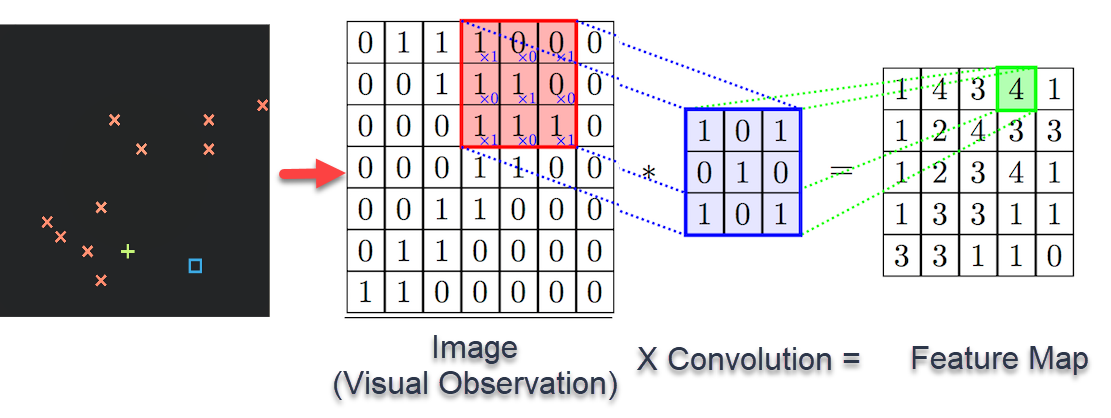(Source: https://subscription.packtpub.com/book/game_development/9781789138139/4/ch04lvl1sec31/convolutional-neural-networks)

(Short project ideas: Check the claims above:
• Garble/permute the rows and columns of the images and see how that affects the training accuracy with convolutional networks (make sure you apply the same permutation to all images!)
• Train a CNN on MNIST digits then do transfer learning on randomly off-centered digits with the convolutional layers fixed and check that you are able to get a similar accuracy to before.
(Link to Colab Notebook where I perform these tests))

(Quick note: There are two forms of pooling, max-pooling, which tests for presence of a feature, and mean-pooling, which tests for sustained presence of a feature. Max pooling may be better if you don't have padding, otherwise it doesn't generally matter which form of pooling you use, as the convolution is capable of spreading a required feature to nearby pixels of a layer.)

In general, the notion that the fundamental operation of the neural network (affine transformations with vectors, convolutions with images) should depend on the "type" of the input data, is an important one.

Once one understands that convolutions are the "fundamental way" of dealing with images, we should simply write convolutional neural networks in the abstract as any standard neural network, i.e. thinking of these "blocks of convolution kernels, plus bias kernels" as the appropriate generalization of "weights, plus biases".Convolutional neural network: each block in the network is a vector of images, i.e. an image with channels.
A very important data "format" to care about is that of a data stream. This is something crucial to any AI that has a concept of time. All the data received by humans is in the form of a data stream, so it is easy to see that processing such data is important when attempting to replicate human tasks.

The natural structure on a data stream is given -- much like the natural structure on an image is given by notions of closeness -- by the flow of time.

Note that simply intuiting the "structure" of the data type doesn't uniquely tell us what the network architecture should be. In fact, the cases of the convolutional and recurrent neural networks aren't even totally analogous -- recurrent neural networks are actually necessary for data streams, because of the input size not being fixed. Nonetheless, principles like parameter sharing are somewhat analogous in each context.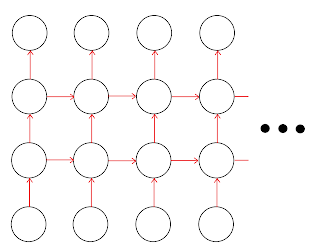Recurrent neural network architecture

The "parameter sharing" that goes on here is that the the parameters of each horizontal slice of the network are the same. Of course, here this parameter-sharing is required by the lack of fixed input size. It's also worth noting how this isn't analogous to convolutional networks, importantly: the information about nearby cells is fed into a layer by neurons in the same layer (as opposed to convolutions, which would have diagonal arrows). Nonetheless, the idea that our processing of a data stream is in some sense "consistent" or "uniform" over time somewhat motivates our understanding of this architecture.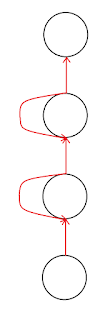Wrapped version of recursive neural network depiction. Input is a data stream.

(In this case, the unwrapped depiction is a better mental model when training the network, as the graph is acyclic, so you can apply backpropagation normally.)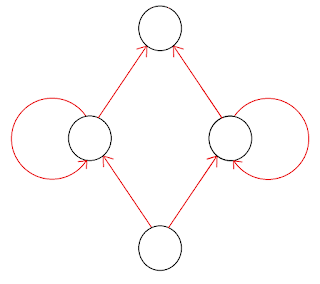"Bidirectional recurrent network", for updating your knwoledge/memory based on new information. This network has two "memory canals", carrying information forward as well as backward. The wrapping suppresses it in the depiction, but the second memory canal feeds into itself in the opposite direction as the data stream.

Data streams: Recurrent neural networks and Turing-completeness

One way to understand recurrent neural networks -- as we did above -- is as the natural algorithm for processing the specific data format called "data stream".

Of course, this isn't really "all there is", The precise structure of the recurrent neural network seems somewhat arbitrary, and it isn't truly completely determined by saying "it's the natural way to process data streams". Some genuinely new structure is seen here, and we should ask for a corresponding universal approximation theorem for recurrent networks.

The question to ask is: what exactly is the new structure seen in RNNs? How, precisely, is it different from standard feedforward networks?

A standard feed-forward network seeks to simulate functions, right? So the "universal approximation theorem" says that a neural network can approximate any function, up to something. So what does a recurrent neural network seek to simulate? What is a "function on a data stream"?

To answer this, we should talk about what exactly a function in the sense of computer science is. A function cannot really "take in a data stream" in computer science. A data stream that has not yet been fully fixed/captured is not a valid input variable, it's not a valid input data type.

What we're trying to simulate isn't really a function -- it's a program. A function is an example of a program, but a more general computer program can actually look at a data stream as it's running and continually update its output based on it. And the analog of universal approximation for programs is Turing-completeness, which RNNs do possess (as proven in [Siegelmann & Sontag, 1992]).

(A short project you can do to test the claim that RNNs indeed simulate programs: check that an RNN tested for a certain length of data input works reasonably well on inputs of different sizes. Can you do this with a CNN?)

You might get the sense that the RNN architecture we've discussed doesn't really feel the same as the way we process streams of data. It seems too generic, like there are more specific tasks we always do in our minds while processing some stream of audio or video. Like we need a better prior, to tell the network the exact nature of what it means to "mix" past and present features.

With the human mind, we have a very specific notion of memory -- specific actions to add and remove things from our memory. This construct is of importance, while watching a movie, holding a conversation, or reading a sentence. It's not just recalled memory, that is stored somewhere and accessed through searching for keywords in the mind, but actively held short-term memory that is particularly relevant in this context. During any of these activities, the human mind will never adopt any other "mixing" mechanism between knowledge from different points in time, that doesn't go through memory.

This is the idea behind Long Short Term Memory (LSTM) networks. Every recurrent "layer" of an LSTM network actually involves the following three computations:

1. The forget filter -- based on current input, the network drops of some elements in the "memory channel" (called the cell state in LSTM)
2. The remember filter -- the network adds some features of the current input to memory at varying intensities.
3. The output filter -- the network allows some of the current memory to pass to output.

I call these "filters" and use language like "dropping elements" and "some features", but it is to be noted that these are really about differences in intensity, and involve multiplying by the output of trainable sigmoid layers that decide how much of your memory/features you want to allow to pass through.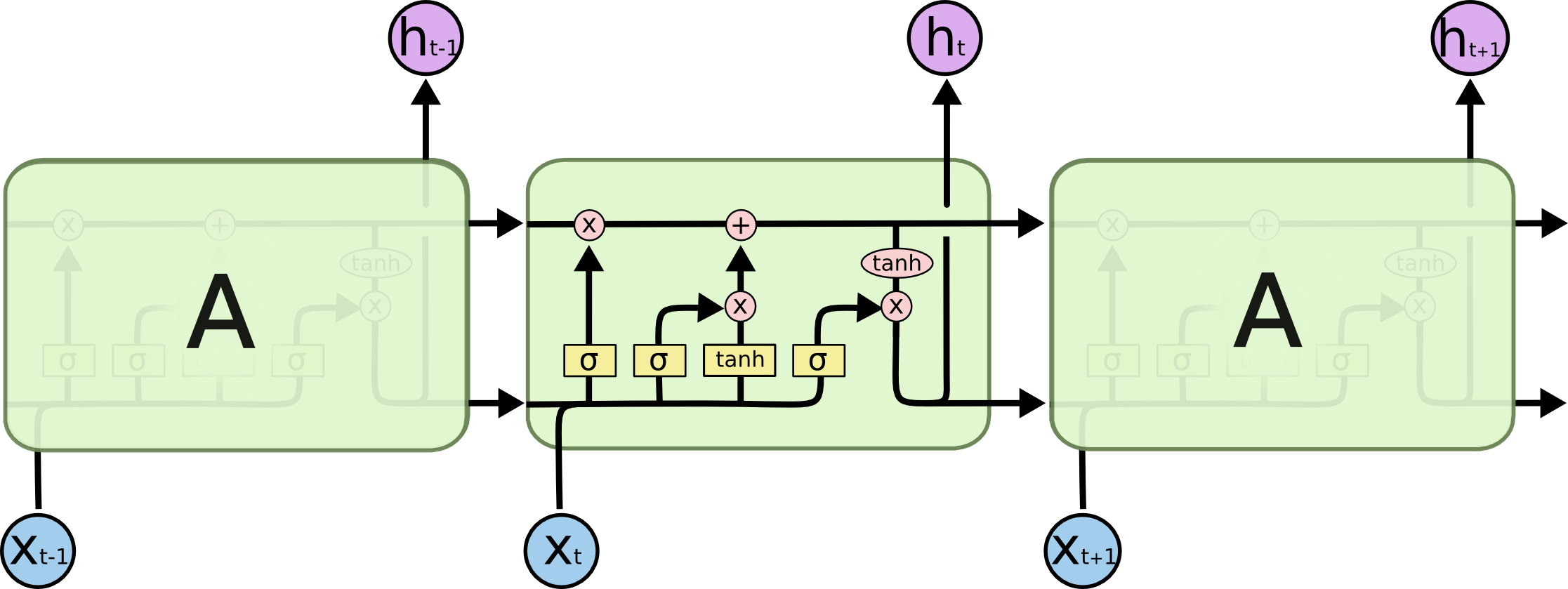Source: Understanding LSTM networks by Christopher Olah

As an exercise, figure out which parts of the network correspond to which of the filters I've mentioned.

(Short project idea: I'm actually not completely sure if I understand whether the bottom channel is needed, i.e. whether its contents must be transfered to the next iteration in the sequence. Experiment with this alternative on standard applications of LSTM and compare the performance.)

Environment interaction: Reinforcement learning

With standard neural networks, feedback is specifically provided in the form of predetermined data and labels that the network is required to predict.

Reinforcement learning can be seen as a generalization of this, where the feedback isn't prepared by something complicated like a human, but instead is the result of dynamic interaction with an environment (or game). The environment typically follows some laws (i.e. the laws of physics, or the laws of a game -- for robotics and game bots respectively), and this automatically generates massive amounts of data, and computes an action's consequences, which act as a generalized notion of data "labels".

A problem is immediately clear with this method: how do you differentiate an environmental response against your network's parameters? This is not a minor technical problem -- differentiation fundamentally requires that you know what happens if you change your parameters a bit.

E.g. suppose you have a network that takes in the current state of a game and outputs a real number between 0 and 1, representing the probability that it tells the agent to "jump". Then you can differentiate each decision with respect to the parameters; however, you cannot differentiate the outcome (win/loss) with respect to the decisions.

The solution to this problem comes from recalling that we are trying to maximize an expected score, so we should be doing some sampling. More formally: we should play around with expectations.

Let $Y$ be the random variable representing the agent's decision (i.e. "jump" or "not") for some given input $x$, with probability function $P(Y|x, \theta)$ where $\theta$ is the network's trainable parameters. Then where $L(x, Y)$ is the environmental loss function, we are interested in minimizing $E(L)$.

\begin{align} {\nabla _\theta }E\left[ {L(Y)} \right] &= {\nabla _\theta }\sum\limits_Y^{} {P(Y)L(Y)} \\ &= \sum\limits_Y^{} {{\nabla _\theta }\left[ {P(Y)} \right]L(Y)} \\ &= \sum\limits_Y^{} {P(Y)\frac{{{\nabla _\theta }P(Y)}}{{P(Y)}}L(Y)} \\ &= \sum\limits_Y^{} {P(Y){\nabla _\theta }\left[ {\log P(Y)} \right]L(Y)} \\ &= {E_Y}\left[ {{\nabla _\theta }\left( {\log P(Y)} \right)L(Y)} \right] \\ \end{align}
So the solution is as follows: sample a large number of gameplays. Now pretend that each decision contributed directly to the victory and optimize them -- encourage all the moves in winning gameplays and all the moves in losing gameplays, i.e. update the parameters by the average value of ${{\nabla _\theta }\left( {\log P(Y)} \right)L(Y)}$ across the sample.

So despite the bizarreness of pretending that every move in a winning play was correct and every move in a losing play was wrong, doing this for a large sample makes incorrect learned features cancel out -- a good move is expected to produce better results when all other moves are averaged out, and a bad move is expected to produce worse results when all other moves are averaged out.

This strategy is known as policy gradients, and is a general technique to deal with non-differentiable feedback.Image source: Andrej Karpathy

(You may notice that this is incredibly inefficient. Indeed, this article only covers the most basic and superficial elements of cognition -- the human brain is capable of reasoning, and of producing a highly abstracted model of the game in its mind, and of transferring intuition from elsewhere onto the game.)

Generation: Generative (matching and adverserial) neural networks

Equipped with the ability to process data, the obvious next step is to get an AI to produce things -- to get an AI to be creative. To come up with art, compositions, original thoughts and ideas. We'll now describe the most elementary of such neural networks, which we will call Generative Neural Networks, while more complicated ideas would exploit some sort of transfer learning.

It's not at all absurd to expect it to be possible for a neural network to generate images of horses that don't look like any horse it's actually seen -- because humans can do that! If you imagine a horse, it's probably not a horse whose image you've seen before, but it nonetheless possesses the features you've identified as common between horses.

The idea behind a generative neural network can be motivated from the following two statistical notions:

• The inverse transform method of generating random variables.

Content generated by a mind can be considered to be a random variable in some fancy space. E.g. if we want to get our neural network to produce (28, 28) digit characters, we're training it into a random variable on the space of (28, 28) images whose support is the images we identify as valid digit characters.

The way that computers typically sample random variables is through the "inverse transform method", which is to start with a uniform random sample and apply $F^{-1}$ to your sample where $F$ is the CDF of the random variable $X$ you want to sample. Your result will be a sample of $X$.Quick explanation of inverse transform method: under the uniform distribution, the probability of getting a value under $u$ is $u$, which under the CDF of $X$ is the probability of getting a value under $F^{-1}(u)$. So you map $u\mapsto F^{-1}(u)$.
So we once again need a function approximator -- to approximate $F^{-1}$.The inputs to the neural network are randomly generated, typically uniformly

• A nonlinear generalization of principal component analysis

Think, e.g. of eigenfaces. If you've ever tried to use eigenfaces to generate realistic faces, you'll notice that your results are just terrible. Much of the information in faces is not so linear and nice -- there's no reason to expect it to be. Illumination and angle are pretty much the only properties that can be expected to vary linearly.

I.e. suppose you have some data that varies as follows:

Then a PCA might give you the pink line as your first principal component, but sampling from the pink line gives you a lot of unphysical outputs, those are the areas where your pink line doesn't intersect the data.

But using PCA to generate samples from a distribution can be understood as taking some random inputs, corresponding to the values of each principal component you want to use, and feeding them through a function, the principal component change-of-basis matrix.

But more generally, replacing this function with something nonlinear allows us to deal with nonlinear models.

OK,so it's clear to us that we need a neural network -- a "generative neural network" -- to construct the inverse CDF. How would one train this network?

Given an initial random guess for the network parameters, what we have is some guessed distribution for "images of horses". And what we really want to do is perturb these parameters to match the distribution of our data.

Well, such an approach is certainly possible -- one could measure some notion of distance from our generated sample distribution and the real distribution and backpropagate this error with each iteration.

Note that we don't actually know the distribution of our data (the red one), so we can't really use something like "the probability of observing this sample given our distribution" as our loss function. Anyway, there are measures of the distance between two distributions that we could use for our error function, such as the maximum mean discrepancy approach, and methods involving moments.

(This approach, generally, is called a Generative Matching Network.)

But being arbitrary is generally disappointing in machine learning, and it's worth asking if there's a way to get the network to learn to discriminate between distributions.

Here's an idea: we could just subjectively tell that the outlier point did not belong to the distribution. We used our human brainsHow about rather than defining a discrimination function, we trained a neural network to tell if a given data point could belong to a distribution? Then this neural network would train our generative neural network, and vice versa.

And this makes sense, right? When we learn to draw an object, we're also simultaneously learning to identify one.

And one could imagine showing off a generative network's results and having people guess if they're real or not (alongside actual real images of course) -- and based on whether they thought it was real, we could use it to train the network. These "people" are precisely what a discriminator network is.

In other words, we have two networks: the generator network, which generates random horse faces from random uniform variables, and the discriminator network, which takes the output of the generator network and some actual images, and figures out if the result is real or not.

If the classification is incorrect, the discriminator network is punished, while if it is correct, the generator network is punished.

This is known as a Generative Adverserial Network.

Making connections: transfer learning

Something of crucial importance to human thought is the ability to make connections between ideas, transfering ideas and results from one area to another, either directly or by "abstracting out" the analogies.

There are many "levels" on which this occurs -- examples follow:
• We don't need 60,000 instances to learn a script -- We can do with 1. It's shocking that we can learn distributional information from a single data point, and suggests that we already have an extraordinarily good prior. And we do -- our prior is continually updated from experience, of course, so this just means we're applying existing knowledge. We already know what features of the script are likely to be important and what can be safely discarded (hint: that accidental wiggle in the straight-ish line is probably noise) away.
• Prerequisites exist. So we depend on "applying" existing knowledge in some sense to learn new things. There's a reason why babies typically don't learn algebraic geometry.
• Making abstract connections between ideas -- Like recognizing that counting apples and counting sticks is the same task (and frivolous details about what an apple is or what a stick is can be lost for our purposes), recognizing that the algebra of kinematic quantities is the same to some extent as the algebra of quantum states (they both form vector spaces) (see Abstraction in mathematicsAbstraction in engineering), or recognizing the analogy between linear transformations of vector spaces and continuous functions of topological spaces (they're both categories).
There are probably multiple different algorithms in the brain that involve analogy and abstraction, and at least some of them (such as the first example above) have to do with learning.

This is the principle behind transfer learning, the idea that one may use the results of existing encoders for processing in other domains (and perhaps the precise encoder used may be fine-tuned for these other purposes).

Examples of transfer learning architectures:

Progressive neural networks

This may be a bit of a disappointing solution to the question "How do brains think abstractly?" Our answer seems to depend on our active, hard-coded choice of what layers and encodings to preserve for our next task. Surely our brains do this somewhat "automatically" -- we don't actively tell our brain "Look, you've seen lines, remember? Well, there's lines here."

Well, maybe sometimes we do. It seems there may be some element of a conscious thought (whatever that means on an algorithmic level) in making analogies when it comes to complex intellectual tasks. But we certainly do not undergo any conscious thought when it comes to something like character recognition.

An architecture that may be analogous to our brain's cognition is that of a progressive neural network -- here, layers are algorithmically transferred from a previous task to another, with transferred parameters frozen. The picture below conveniently helps us make the analogy to "lateral thinking".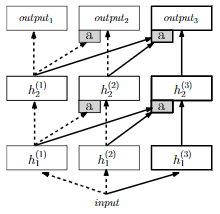Source: arXiv:1606.04671

This architecture is capable of (and is about) choosing the sources of information that are most effective for the required task, but what suggests to me that this isn't what our brain does exactly is the parameter wastefulness and increasing level of model complexity (trainable parameters), which doesn't seem to be analogous with our method of learning, which seems to be more or less symmetric between learned ideas.Hard parameter sharing. Source: Sebastian RuderSoft parameter sharing. Source: Sebastian Ruder